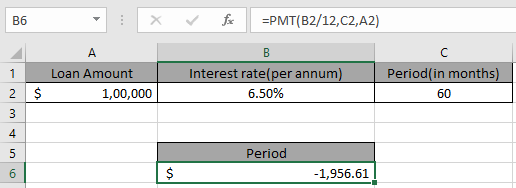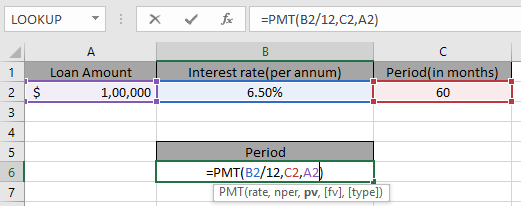# How to use the PMT function in ExcelIn this article, we will learn How to use the PMT function in Excel.

What is this PMT function used to calculate a loan ?

Excel let’s a person find monthly installment on a loan amount using the function having principle amount or loan amount, interest rate per month and the period of payment.

PMT Function in Excel

The function calculates the payment for a loan based on constant payments and a constant interest rate.

Syntax:

 =PMT (rate, nper, pv, [fv], [type])

rate The interest rate per period.

per - The period for which the interest rate is calculated.

nper - The total number of payments.

pv - The present value, the total amount that a series of future payments is worth now.

fv - [optional] The future value, or a cash balance you want after the last payment is made. Defaults to 0 (zero).

type -[optional] The timing of the payment, either at the beginning or end of the period. Numbers 0 or 1 represent the payment date. The number 0 represents payment at the end of the period, and the number 1 represents payment at the beginning of the period. The default (empty argument) is 0. The calculation is at the end of the period.

Example :

All of these might be confusing to understand. Let's understand how to use the function using an example. Consider a scenario, John has taken a loan of \$100,000 from a bank at 6.5% per year interest in a 5 years period. He needs to find out his monthly payment to the bank paying his loan.

So he has the following details in Excel.Use the formula in B6 cell

 =PMT(B2/12,C2,A2)You must be wondering why Interest rate is divided by 12 as  Applying the formula in the cell.John has to pay \$1,956 per month. You must be wondering why this amount shown here is negative. The loan amount is positive which is credited to John. He has to pay that amount which is to be debited.

You can use minus sign(-) in the formula to get the result in positive sign(+)

Use the alternative formula for signs

 =PMT(B2/12,C2, -A2)

Here are some observational notes using the PMT function in Excel

Notes:

1. The function returns #NUM! Error  when:
1. The given rate value is less than or equal to -1.
2. The given nper value is equal to 0.
2. The function returns #VALUE! error when any of the arguments provided are non-numeric.
3. When calculating monthly or quarterly payments, we need to convert annual interest rates or the number of periods to months or quarters.
4. If we wish to find out the total amount that was paid for the duration of the loan, we need to multiply the PMT as calculated by nper.

Hope this article about How to use the PMT function in Excel is explanatory. Find more articles on calculating values and related Excel formulas here. If you liked our blogs, share it with your friends on Facebook. And also you can follow us on Twitter and Facebook. We would love to hear from you, do let us know how we can improve, complement or innovate our work and make it better for you. Write to us at info@exceltip.com.

Related Articles :

How to use the MIRR function in excel : returns the Modified interest rate of return for the financial data having Investment, finance rate & reinvestment_rate using the MIRR function in Excel.

How to use the XIRR function in excel : returns the Interest rate of return for irregular interval using the XIRR function in Excel

Excel PV vs FV function : find Present Value using PV function and future value using FV function in Excel.

How to use the RECEIVED function in excel : calculates the amount which is received at maturity for a bond with an initial investment (security) and a discount rate, there are no periodic interest payments using the RECEIVED function in excel.

How to use the NPER function in excel : NPER function to calculate periods on payments in Excel.

How to use the PRICE function in excel : returns the price per \$100 face value of a security that pays periodic interest using the PRICE function in Excel.

Popular Articles :

How to use the IF Function in Excel : The IF statement in Excel checks the condition and returns a specific value if the condition is TRUE or returns another specific value if FALSE.

How to use the VLOOKUP Function in Excel : This is one of the most used and popular functions of excel that is used to lookup value from different ranges and sheets.

How to use the SUMIF Function in Excel : This is another dashboard essential function. This helps you sum up values on specific conditions.

How to use the COUNTIF Function in Excel : Count values with conditions using this amazing function. You don't need to filter your data to count specific values. Countif function is essential to prepare your dashboard.

Terms and Conditions of use

The applications/code on this site are distributed as is and without warranties or liability. In no event shall the owner of the copyrights, or the authors of the applications/code be liable for any loss of profit, any problems or any damage resulting from the use or evaluation of the applications/code.